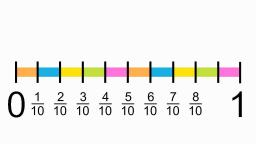# Denominator 2883

Write a fraction with denominator 200 that is greater than 0.39 and less than a two-fifths fraction. Write its numerator x.

x =  79

### Step-by-step explanation:Did you find an error or inaccuracy? Feel free to write us. Thank you!

Tips for related online calculators
Need help calculating sum, simplifying, or multiplying fractions? Try our fraction calculator.